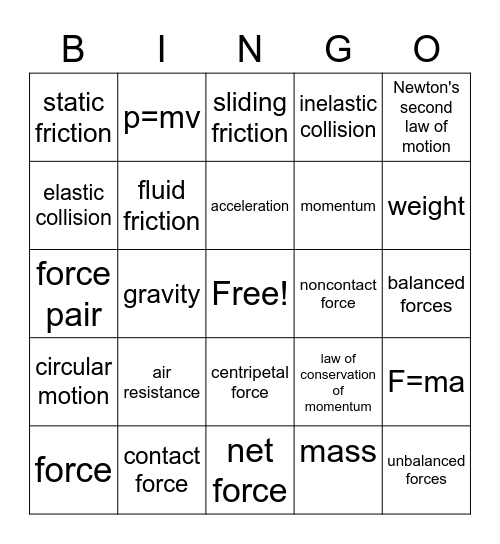# Newton's Laws of MotionThis bingo card has a free space and 29 words: force, friction, gravity, inertia, static friction, sliding friction, fluid friction, air resistance, noncontact force, contact force, balanced forces, unbalanced forces, net force, mass, weight, Newton's first law of motion, centripetal force, circular motion, Newton's second law of motion, force pair, law of conservation of momentum, Newton's third law of motion, momentum, elastic collision, inelastic collision, p=mv, F=ma, acceleration and 9.8 m/s/s.

## Play Online